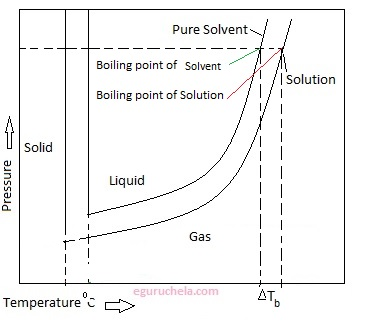# Elevation of boiling point

Boiling-point elevation describes the phenomenon that the boiling point of a liquid (a solvent) will be higher when another compound is added, meaning that a solution has a higher boiling point than a pure solvent.

The extent of boiling-point elevation can be calculated by applying Clausius–Clapeyron relation and Raoult's law together with the assumption of the non-volatility of the solute. The result is that in dilute ideal solutions, the extent of boiling-point elevation is directly proportional to the molal Concentration of the solution according to the equation

ΔTb = Kb • bB

where

ΔTb, the boiling point elevation, is defined as Tb (solution) - Tb (pure solvent).

Kb, the ebullioscopic constant, which is dependent on the properties of the solvent. It can be calculated as:

Kb = RTb2M/ΔHv, where

R is the gas constant,

Tb is the boiling temperature of the pure solvent [in K],

M is the molar mass of the solvent,

ΔHv is the heat of vaporization per mole of the solvent.

bB is the molality of the solution, calculated by taking dissociation into account since the boiling point elevation is a colligative property, dependent on the number of particles in solution.

Example:

The boiling point of salted water is higher than the boiling point of pure water.### Factors affect boiling point

The boiling point of a liquid depends on following factors:

temperature,

atmospheric pressure and

the vapor pressure of the liquid.